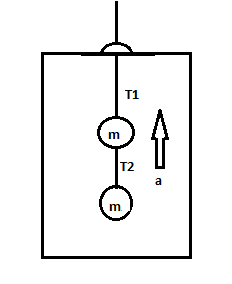# Two blocks each of mass m = 3.50 kg are fastened to the top of an elevator as in figure. (a) If...

## Question:

Two blocks each of mass m = 3.50 kg are fastened to the top of an elevator as in figure.

(a) If the elevator has an upward acceleration a = 1.60 {eq}m/s^2 {/eq}, find the tensions {eq}T_1 {/eq} and {eq}T_2 {/eq} in the upper and lower strings.

(b) If the strings can withstand a maximum tension of 85.0 N, what maximum acceleration can the elevator have before the upper string breaks?## Newton's Second Law:

As per Newton's second law, the net force acting on the body in any direction is equal to the multiplication of mass of the matter of the body and the acceleration of the body in that particular direction.

## Answer and Explanation:

Become a Study.com member to unlock this answer! Create your account

Given Data:

• The mass of the each block is: {eq}m = 3.5\;{\rm{kg}} {/eq}.
• The acceleration of the elevator is: {eq}a = 1.6\;{{\rm{m}}...

See full answer below.

#### Learn more about this topic:Force: Definition and Types

from

Chapter 5 / Lesson 5
81K

Force is everywhere and it comes in a variety of sizes, directions, and types. In this video lesson, you'll identify force as well the different types of force that objects may experience.